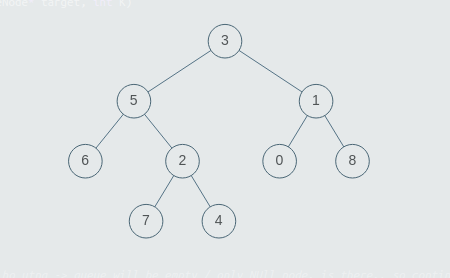# Print Nodes at Distance K From a Given Node

Posted: 6 Nov, 2020
Difficulty: Hard

## PROBLEM STATEMENT

#### Note:

``````1. A binary tree is a tree in which each node has at most two children.
2. The given tree will be non-empty.
3. The given tree can have multiple nodes with the same value.
4. If there are no nodes in the tree which are at distance = K from the given node, return an empty list.
5. You can return the list of values of valid nodes in any order. For example if the valid nodes have values 1,2,3, then you can return {1,2,3} or {3,1,2} etc.
``````
##### Input Format:
``````The first line of the input contains an integer T, denoting the number of test cases to run.

The first line of each test case will contain the values of the nodes of the tree in the level order form ( -1 for NULL node). Refer to the example below for further explanation.

The second line of each test case contains the value of the target node.

The third and the last line of each test case contains the integer K denoting the distance at which nodes are to be found.

Example:

Consider the binary tree:
````````````The input for the tree depicted in the above image would be :

3
5 1
6 2 0 8
-1 -1 7 4 -1 -1 -1 -1
-1 -1 -1 -1

Explanation :
Level 1 :
The root node of the tree is 3

Level 2 :
Left child of 3 = 5
Right child of 3 = 1

Level 3 :
Left child of 5 = 6
Right child of 5 = 2
Left child of 1 = 0
Right child of 1 = 8

Level 4 :
Left child of 6 = null (-1)
Right child of 6 = null(-1)
Left child of 2 = 7
Right child of 2 = 4
Left child of 0 = null (-1)
Right child of 0 = null (-1)
Left child of 8 = null (-1)
Right child of 8 = null (-1)

Level 5 :
Left child of 7 = null (-1)
Right child of 7 = null (-1)
Left child of 4 = null (-1)
Right child of 4 = null (-1)

The first not-null node (of the previous level) is treated as the parent of the first two nodes of the current level. The second not-null node (of the previous level) is treated as the parent node for the next two nodes of the current level and so on.

The input ends when all nodes at the last level are null (-1).
``````
##### Output Format :
``````For each test case, print the values of all nodes at distance = K, from the given target node.
``````

#### Note:

``````You do not need to print anything, it has already been taken care of. Just implement the given function.
``````
##### Constraints:
``````1 <= T <= 100
1 <= N <= 3000
0 <= K <= 3000
0 <= nodeValue <= 3000

Where nodeValue donates the value of the node.

Time Limit: 1 sec
``````Approach 1
• Create a map to store the parent of each node in the tree, Traverse the tree recursively (via depth-first search), at each step if the current node is not NULL. Store its parent in the map, then traverse the left and right subtree.
• Now assume that the given node is the root of the tree. In such a case, we can simply run a breadth-first search from the root, and track the current level of the tree. When the level = ‘K’, then the current nodes in the queue will be our answer.
• But the problem is if the target node is not the root, then we can’t travel to every node with only left and right pointers. So here the stored parents will help us.
• Observe that in a binary tree a node can be connected to maximum 3 other nodes ie. left child, right child, and the parent. We have left and right pointers, and we have also stored the parent node.
• Now simply start BFS from the target node, and for each node which is at front of the queue, push its left child, right child and parent node(provided they are not null).
• When the level of BFS reaches ‘K’, break the BFS and store all the values of nodes in the queue to an array and return it.